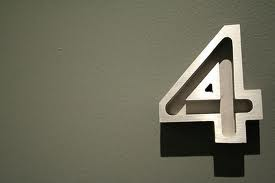# Do I Know a Number Other Than 4?When $(4444)^{4444}$ is written in decimal notation, the sum of its digits is $A$. Let $B$ be the sum of the digits of $A$. Find the digital sum of $B.$

(Note: A digital sum means you take the sum of the digits, and then if more than one digit remains, take the sum again, and keep repeating until only one digit remains.)

×